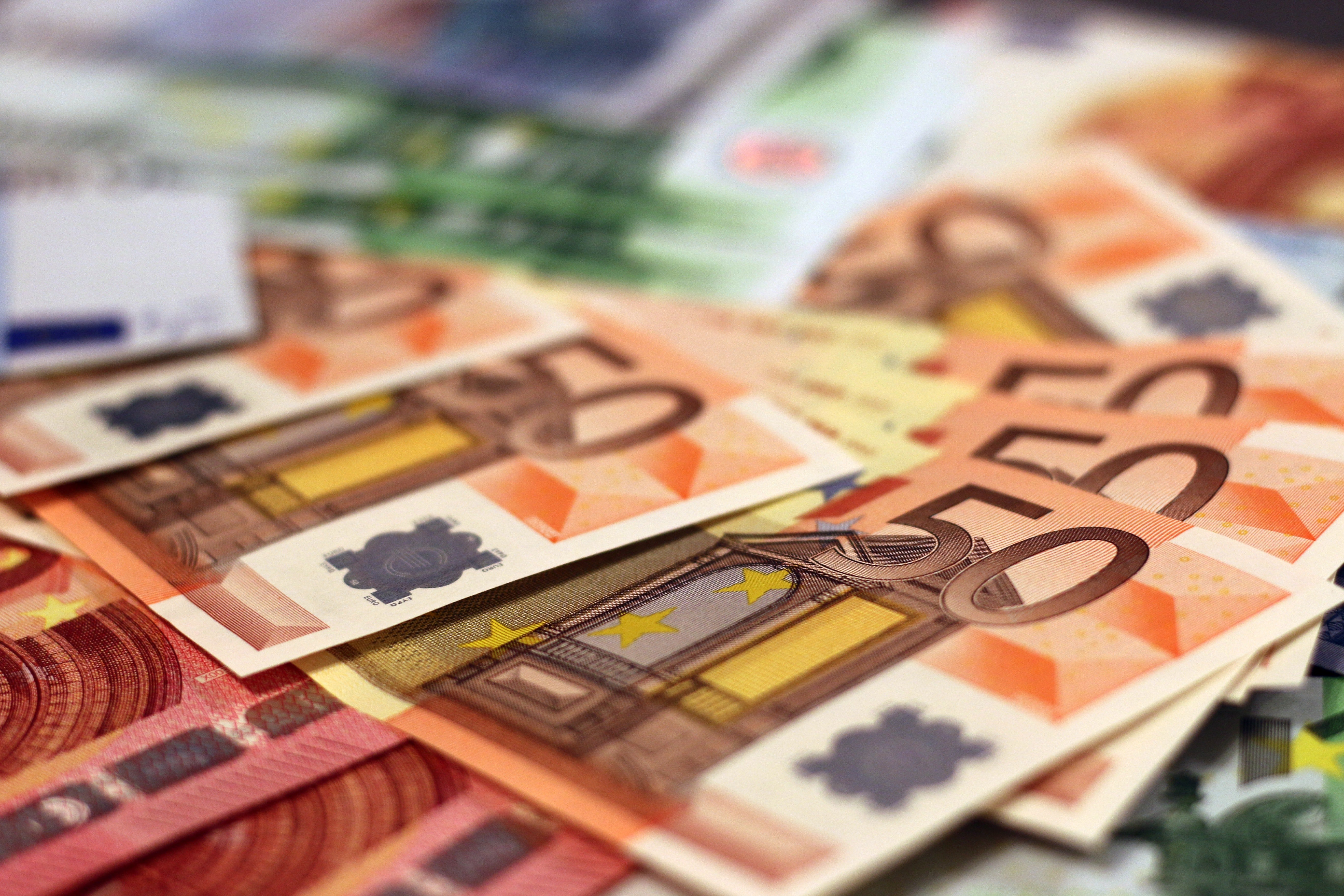﻿ What Is A PEG Ratio? - FXCM South Africa# What Is A PEG Ratio?

The PEG ratio is a popular method of determining the relative value of a company's stock taking into account not only its price and earnings but also its growth rate. While the price-to-earnings (P/E) ratio is arguably the most common method of determining a stock's worth relative to other companies, it doesn't factor in the company's growth prospects, which can make a big difference in its value. The PEG (price to earnings to growth) ratio seeks to correct this deficiency.

Simply put, the PEG ratio is the price-to-earnings ratio divided by the company's estimated growth rate, usually over the next 12 months (using a longer period of time is generally less accurate), as follows:

PEG ratio = Price/earnings/growth rate

The idea of the PEG ratio is generally credited to Mario Farina, the author of A Beginner's Guide To Successful Investing In The Stock Market, published in 1969. It gained more popularity thanks to Peter Lynch, the legendary former manager of the Fidelity Magellan Fund, who argued that a company's P/E ratio should always equal its growth rate, meaning that it's PEG ratio equals exactly 1.0. If it doesn't, that means it's not properly valued by the market.

As Lynch wrote in his 1989 book One Up on Wall Street:

"The P/E ratio of any company that's fairly priced will equal its growth rate. If the P/E of Coca-Cola is 15, you'd expect the company to be growing at about 15 percent a year, etc. But if the P/E ratio is less than the growth rate, you may have found yourself a bargain. A company, say, with a growth rate of 12 percent a year...and a P/E ratio of 6 is a very attractive prospect. On the other hand, a company with a growth rate of 6 percent a year and a P/E ratio of 12 is an unattractive prospect and headed for a comedown...In general, a P/E ratio that's half the growth rate is very positive, and one that's twice the growth rate is very negative."

The goal of the PEG ratio is to help investors spot stock market bargains while avoiding stocks that are overpriced. If two stocks have identical P/E ratios but have vastly different growth rates, one stock may be undervalued while the other may be overpriced.

For example, Company A and Company X both have a P/E ratio of 10, but Company A expects to grow earnings by 20% in the following year while Company X expects to grow by only 5%. Clearly, based on their respective P/E ratios, Company A is undervalued while Company X is overpriced, even though both have the same P/E ratio. But by factoring in their growth prospects—i.e., by calculating their respective PEG ratios—we get a clearer picture of their relative worth.

In this example, Company A's PEG ratio is 0.5% (10 divided by 20 = 0.5), while Company X's is 2.0 (10 divided by 5 = 2.0). Generally speaking, the lower the PEG ratio below 1.0, the greater the bargain, as Lynch said. Conversely, the higher the PEG ratio above 1.0, the more overpriced the stock is.

As general rule, a stock's PEG ratio should be less than 1, which would indicate that it's underpriced, while a PEG ratio greater than one might mean that the stock is overvalued, assuming the growth rate estimate is accurate.

Like most other ways of valuing stocks and estimating their future performance, the PEG ratio has both advantages and disadvantages.

The biggest advantage the PEG ratio has its sheer simplicity and ease of computing, making for a relatively simple apples-to-apples comparison between stocks. Plus, it goes one step beyond the P/E ratio, adding the important element of future growth.

However, like most other indicators, it isn't perfect, and is only as accurate as the data put into the equation—garbage in, garbage out, as the saying goes. While the price of a stock is easily known by what it's trading at, earnings (assuming the analyst is using forward earnings versus past earnings) and growth rates are both merely estimates.

Therefore, if the estimates are flawed or prove to be inaccurate, then the PEG ratio will also be wrong. However, the same argument could be made about many other tools that securities analysts use, many of which are built on forecasts—if not outright guesses—and not hard data.

The PEG ratio is also generally more appropriate for estimating the value of smaller, younger and faster-growing companies than more mature companies. The older companies may not grow as fast but have other benefits that bolster their values and future prospects, such as dividends, large corporate war chests, the ability to borrow money cheaply and easily, and proven long-term sustainability.

The PEG ratio also doesn't measure other factors that can influence a stock's price, such as the company's debt load. However, the ratio does not purport to be a be-all-and-end-all value indicator. It's just another tool in the analyst's arsenal.

## Summary

The PEG ratio measures a stock's relative value by factoring in its growth rate. The ratio is simply the company's price-to-earnings (P/E) ratio divided by its estimated growth rate. As a rule of thumb, a company's PEG ratio should equal 1.0, meaning that it's P/E ratio exactly equals its estimated earnings growth rate. Generally speaking, investors should seek out stocks whose PEG ratio is less than 1.0, meaning the stock is undervalued, while a PEG ratio greater than 1.0 would indicate that it's overpriced.